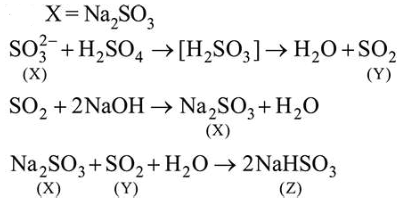# Reaction of an inorganic sulphite X withQuestion:

Reaction of an inorganic sulphite $\mathrm{X}$ with dilute $\mathrm{H}_{2} \mathrm{SO}_{4}$ generates compound Y. Reaction of $Y$ with $\mathrm{NaOH}$ gives $X$. Further, the reaction of $\mathrm{X}$ with $\mathrm{Y}$ and water affords compound Z. Y and Z, respectively, are:

1.  $\mathrm{SO}_{2}$ and $\mathrm{Na}_{2} \mathrm{SO}_{3}$

2.  $\mathrm{SO}_{3}$ and $\mathrm{NaHSO}_{3}$

3. $\mathrm{SO}_{2}$ and $\mathrm{NaHSO}_{3}$

4. $\mathrm{S}$ and $\mathrm{Na}_{2} \mathrm{SO}_{3}$

Correct Option: , 3

Solution: